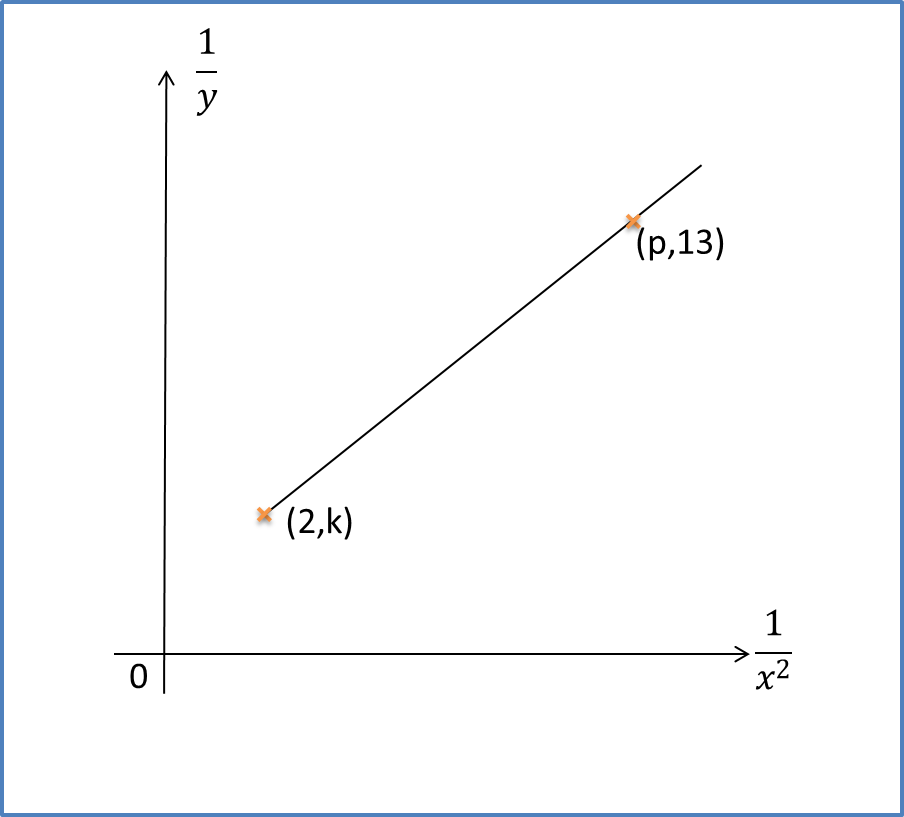\

# SPM Practice 2 (Question 4 & 5)

Question 4:
The diagram shows part of the straight line graph obtained by plotting $\frac{y}{x}$ against $\sqrt{x}$.Given its original non-linear equation is $y=px+q{x}^{\frac{3}{2}}$.  Calculate the values of p and q.

Solution:Question 5:
The diagram below shows the graph of the straight line that is related by the equation $\frac{x}{y}=\frac{2}{x}+3x$.Find the values of p and k.

Solution: